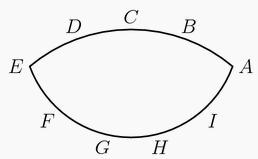PlaneGeometry AIME
2015

Problem - 58

Point $A,B,C,D,$ and $E$ are equally spaced on a minor arc of a cirle. Points $E,F,G,H,I$ and $A$ are equally spaced on a minor arc of a second circle with center $C$ as shown in the figure below. The angle $\angle ABD$ exceeds $\angle AHG$ by $12^\circ$. Find the degree measure of $\angle BAG$.